×

Search anything:

# Here documents in Linux

#### Linux Software EngineeringGet this book -> Problems on Array: For Interviews and Competitive Programming

A here document is a special code block with multi-line strings that can be redirected to the command script or interactive program. In this article, we learn about here documents in Linux.

1. Introduction.
2. Syntax.
3. Substituting parameters and commands.
4. Function arguments.
5. Piping and redirection.
6. Here documents with SSH
7. Summary.
8. References.

## Introduction.

A here document uses a form of I/O redirection to feed a command list to an interactive program or command such as cat, FTP, tee, wall.

An example, we wrote a program that uses small enough inputs, instead of storing this inputs in another text file, we can store the code and its input in the same file using here documents.

Another example, we want to execute multiple commands on a remote system over SSH, however we don't want to do this interactively step by step. For this, we place all the SSH commands in a here document on lines and execute the script once.

## Syntax.

We use the << character followed by a delimiter which we also use to close the here document.

``````command << delimiter
.. .. ..
.. .. ..
delimiter
``````

First, we define a command, such as FTP, cat. This is followed by the << character and a delimiter.

Commonly used strings are EOF or END although we can use any we find preferable.
Inside the here block, we can have anything from strings, variables, inputs, commands.

Finally, we have a delimiter, this should always be the last line and it should not have white space in front of it.

An example of reading input from a source:

``````cat << EOF
An example of here documents.
These lines will be printed.
EOF
``````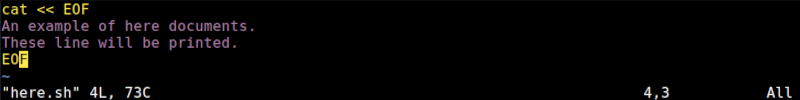From the above, the redirection tells the shell to read from the current delimiter until a similar delimiter is encountered. In this case, our delimiter is EOF, so until it is encountered the lines between will be printed out.

## Substituting parameters and commands.

In here-documents/heredoc we can specify parameters using variables. For example to pint the current working directory we can pass parameters as follows:

``````cat << EOF
EOF
``````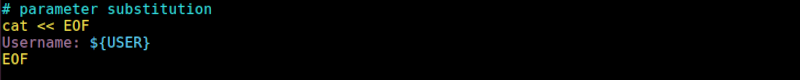In the output, the variable USER is replaced with the actual value of the USER parameter in the terminal. This will change dynamically based on the parameter's value.

Normally, when the delimiter is unquoted, it translates all commands and variables before passing the here documents to the command. If we place the delimiter between quotes, we have the result:

``````cat << "EOF"
We are: \$(whoami)
Contents of home: \$(ls ~/)
EOF
``````When we execute this, there will be no translation done by the shell.

We can also specify commands in a here document which are then substituted with their actual output during execution. An example:

``````cat << EOF
We are: \$(whoami)
The contents of home: \$(ls /)
EOF
``````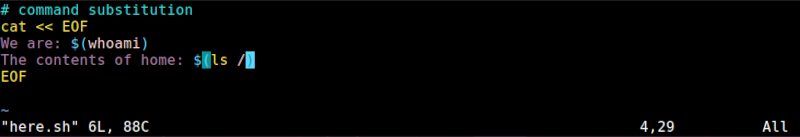## Function arguments.

Assuming we have the following function:

``````# arguments
profile(){
echo "This is a \${name} \${model} from \${year}"
}
``````

The above function takes three arguments the name, model, and year. Normally when we execute this function the function will wait for the arguments interactively.
Alternatively, we could place all this in a here document and pass the value at once as follows:

``````profile << EOF
Toyota
Corolla
1994
EOF
``````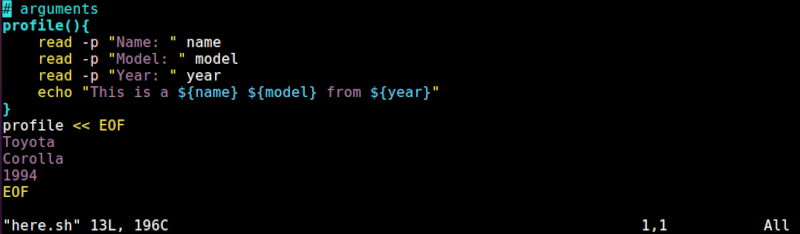## Piping and redirection.

We can also redirect output to a file by using the > and >> characters. The former replaces a file if it exists or adds new content to an empty file, the latter appends content to an already existing file:
An example:

``````cat << EOF > file.txt
The current working directory is: \$PWD
You are logged in as: \$(whoami)
EOF
``````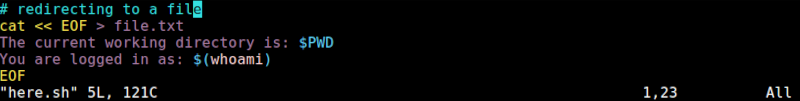We can also pipe command output, for example, let's search and replace using perl:

``````#!/bin/bash
cat << EOF
This is original content
EOF

echo

cat << 'EOF' | perl -pe 's/original/replaced/g;'
This is original content
EOF
``````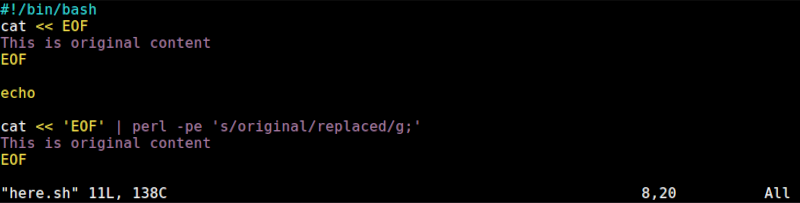In the above example, we have searched for the text original and replaced it with the text replaced.

To use comments in heredocs, for example for a multiline comment we write:

``````#!/bin/bash
<<multiline_comment
This is a mutli line comment
The script replaces text
multiline_comment
``````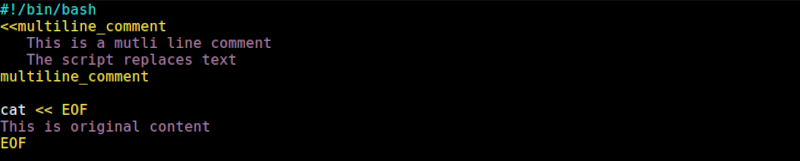The text between the multiline_comment will not be printed as output.

## Here documents with SSH:

Assuming we want to execute multiple commands on a remote system via SSH without using an interactive terminal. For this, we need to write these commands in a remote ssh terminal to perform a backup, copy files and create a firewall rule:
The session would be as follows:

``````\$ ssh user@host uptime > time.txt
\$ ssh user@host date
\$ ssh user@host cal
``````

It is much easier to use a here document to achieve the same result easily. We would write a here document as follows:

``````ssh -T user@host.com << EOF
uptime > time.txt
date
cal
EOF
``````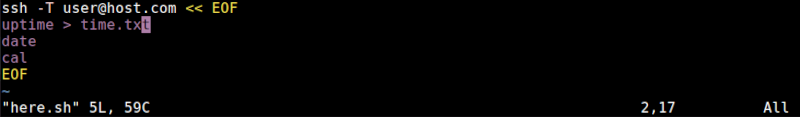## Summary.

Here documents are also referred to as heredocs, they are a type of redirection allowing us to pass multi-line inputs to a command such as ssh, FTP.

#### Erick Lumunge

Erick is a passionate programmer with a computer science background who loves to learn about and use code to impact lives positively.

Here documents in Linux